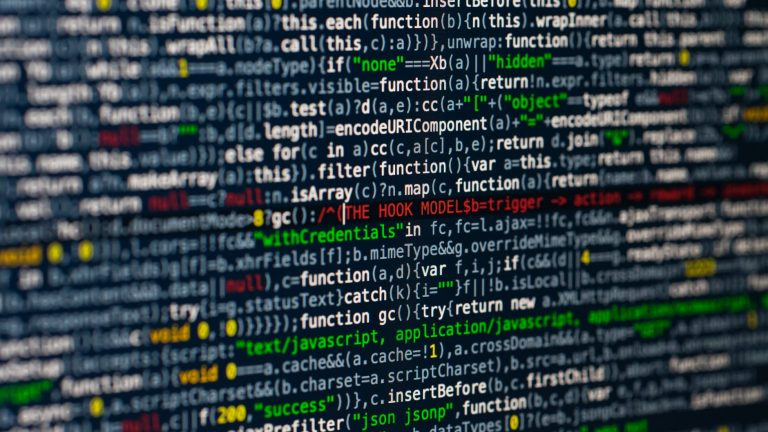## Alternative to the Deprecated PHP “each” Function

By Rajarshi Banerjee# Alternative to the Deprecated PHP “each” Function

Recently I have encountered an issue with my old codes. Those were running just fine earlier. Issue occurs while my server updated its PHP version to 7.2.

Actually, this is common, with up-gradation to server configurations some old functions get deprecated, and in my case, it was PHP “each” function.

With PHP version 7.2 “each” function got deprecated and hence my old codes throwing warnings.

I have to modify my codes and replace the use of “each” with relevant alternatives. Luckily my old codes have a modular structure and most of my logic is bound into some functions, hence I have to change only a few lines of code to remove the warnings.

Here I am giving some examples of the use of “each” function and alternative way to replicate them with PHP 7.2 compatible codes.

### EACH usage:

(is_array(\$data)) ? list(\$data, \$params) = each(\$data) : \$params = NULL;

### Alternative:

(is_array(\$data)) ? list(\$data, \$params) = \$this->altEach(\$data) : \$params = NULL;

function altEach(&\$data)

{

\$key = key(\$data);

\$ret = (\$key === null)? false: [\$key, current(\$data), ‘key’ => \$key, ‘value’ => current(\$data)];

next(\$data);

return \$ret;

}

### EACH usage:

for(reset(\$data); \$keyvalue = each(\$data);) {…}

### Alternative:

foreach (\$data as \$key => \$val) {

\$keyvalue = [\$key, \$val];

}

### EACH usage:

\$data = array(‘arrayInp’ => array(), ‘intInp’ => 2, ‘otherInp’ => null);

\$output = each(\$data);

### Alternative:

\$data = array(‘arrayInp’ => array(), ‘intInp’ => 2, ‘otherInp’ => null);

\$output = [key(\$data), current(\$data)];

### EACH usage:

\$i = 0;

reset(\$data);

while( (list(\$key, \$value) = each(\$data)) || \$i < 30 ) {

\$i++;

}

### Alternative:

reset(\$data);

for (\$i = 0; \$i < 30; \$i++) {

\$key = key(\$data);

\$value = current(\$data);

next(\$data);

}

Hope that will help.Rajarshi Banerjee Subscriber